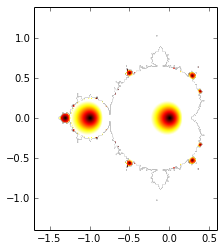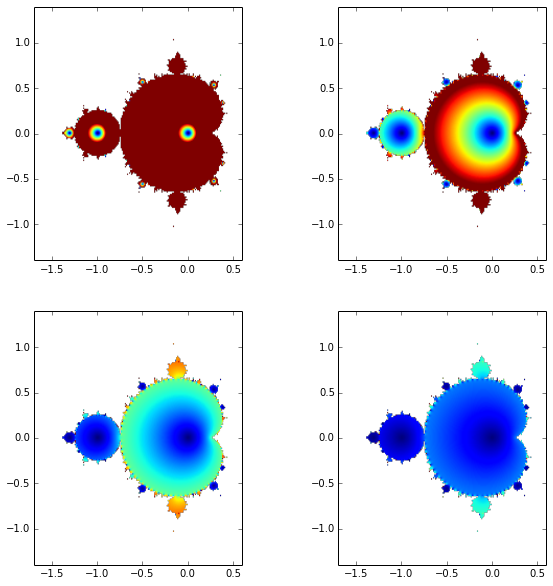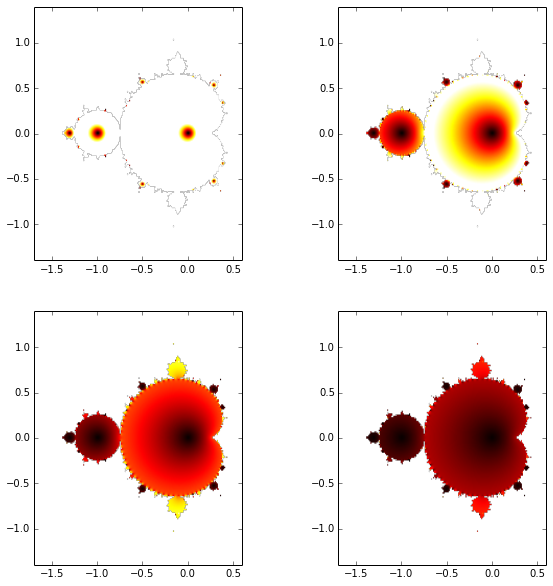The Mandelbrot fractal

The Mandelbrot fractal is a well known mathematical object, foremost for its beauty. You can read about it in Wikipedia or try a related Google image search. There even are hypnotic videos made about it on YouTube.

Anyhow, in this post, we're going to explore how to draw it, with the help of a couple of Python commands.

In :
# necessary imports
%matplotlib inline
from pylab import linspace, zeros, imshow, complex64, subplot, figure

Below, we're defining the function that will determine if a complexe number is part of the Mandelbrot set, or not.

Simply said, the function below, when given a complex number $z$ as an input, can grow large or stay small. It consists of a loop repeatedly squaring the previous value.

To determine if $z$ is part of the Mandelbrot set, we just compare its modulus to a given value (say 1000, for example).

In :
def f(z, n=100):
f = 0
for i in range(n):
f = f**2 + z
return f

The function above is evaluated on a grid defined in the next cell. The chosen number of points in the grid makes the loop fast or slow, depending on how big it is.

In :
num = 200
grid_x = linspace(-1.7, 0.6, num=num)
grid_y = linspace(-1.4, 1.4, num=num)

We can then initialize the matrix that will hold the complex-type data return by the function f.

In :
data = zeros((grid_x.size, grid_y.size), dtype=complex64)

Below comes the loop that evaluates the function f on the previously defined grid. This is the part of the program that takes time.

In :
%%time
for ind_x, x in enumerate(grid_x):
for ind_y, y in enumerate(grid_y):
data[ind_x, ind_y] = f(x + 1j * y)
Wall time: 20.2 s
-c:4: RuntimeWarning: overflow encountered in cdouble_scalars
-c:4: RuntimeWarning: invalid value encountered in cdouble_scalars

Finally, we can plot the computed boundaries of the Mandelbrot set with the following code.

In :
extent = (min(grid_x), max(grid_x), min(grid_y), max(grid_y))
imshow(abs(data.T)**2 < 1000, cmap='gray',  extent=extent)
Out:Another fun thing to do is to interactively explore the images resulting from plotting the modulus of the data using the latest IPython widget machinery. Unfortunately, this does not render to the static view.

In :
from IPython.html.widgets import interactive
In :
def plot_vmax(vmax=0.2, cmap='hot'):
imshow(abs(data.T), cmap=cmap,  vmax=vmax, extent=extent)
In :
interactive(plot_vmax,
vmax=(0.1, 2, 0.05),
cmap=('hot', 'jet'))Based on the previous explorations, I the following two nicely colored figures from the data.

In :
figure(figsize=(10, 10))
for sub, vmax in enumerate([0.1, 0.45, 1.05, 2.]):
subplot(2, 2, sub + 1)
plot_vmax(vmax, 'jet')In :
figure(figsize=(10, 10))
for sub, vmax in enumerate([0.1, 0.45, 1.05, 2.]):
subplot(2, 2, sub + 1)
plot_vmax(vmax, 'hot')This post was entirely written using the IPython notebook. You can see a static view or download this notebook with the help of nbviewer.